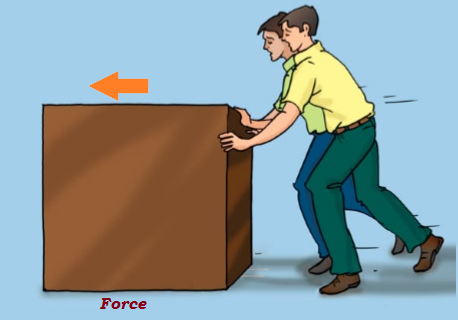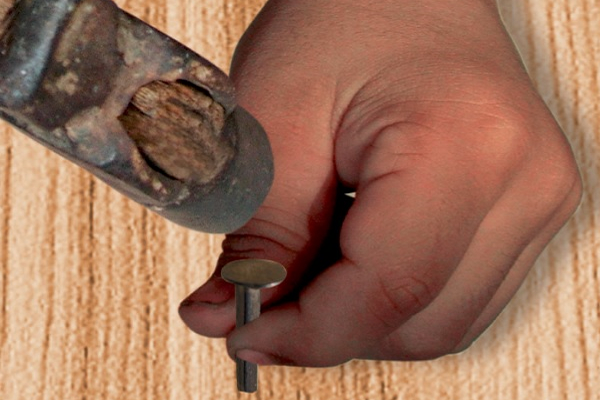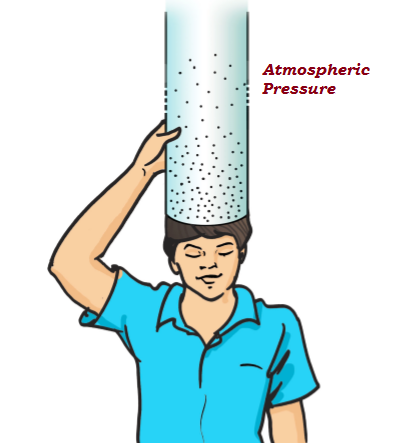# Physics - Force and Pressure

## Introduction

• When an object is either pushed or a pulled is known as a force.

• Motion, generated in an object, is because of an action of a force.• The applied force makes the table move in a given direction.

• The strength of a force is commonly expressed by the magnitude.

• Force also has direction; likewise, if the magnitude or direction changes, it directly affects the force.

• If the force is applied in the direction opposite to the direction of motion, then it results in a decrease in the speed of the object.

• If an object is in motion, then external force may change in the state or direction of motion of that object.

• The state of motion of an object is explained by its speed and the direction of motion.

• The state of ‘rest’ of an object is considered to be the zero speed, as −

• An object cannot move by itself.

• An object cannot change its speed by itself.

• An object cannot change its direction by itself.

• An object cannot change by itself.

• A force may make an object move from rest.

• A force may change the speed of a moving object.

• A force may change the direction of a moving object.

• A force may change the shape of an object.

• The force caused by the action of muscles is known as the muscular force.

• Some force, decreases the speed of a moving object, is known as ‘friction.’ E.g. moving wheel on road; once the source of force stops working, then wheel stops because of friction.

• The force applied by a charged body on another charged or uncharged body is known as ‘electrostatic force.’

• Objects or things that fall towards the earth, as earth pulls it towards itself; this force is known as the force of gravity or gravity.

• The force of gravity is applicable on all objects. In fact, every object in this universe, irrespective of its size and shape, exerts some force on every other object. It happens only because of the ‘gravitational force.’

## Pressure

• The force, applied on a unit area of a surface is known as pressure (Pressure = force/area on which it acts).

• If the area is smaller, then the pressure on a surface would be greater; e.g. this is the reason that the area of one end of a nail is pointed (to exert sufficient pressure) and other end is bigger (as shown in the image given below).• This envelop of air is known as the atmosphere that extends up to many kilometers above the surface of the earth.

• The pressure exerted by the air is known as atmospheric pressure.

• The pressure inside our bodies is exactly equal to the atmospheric pressure and annuls the pressure acting from outside (see the image given below).• Liquids and gases also exert pressure on the walls of their respective containers.